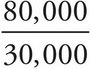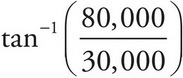# AP Physics 1 Question 393: Answer and Explanation

### Test Information

Question: 393

1. A 2000 kg truck traveling north at 40.0 m/s collides with a 1500 kg car traveling west at 20.0 m/s. If the two vehicles lock bumpers and stick together, what is the angle of the velocity after impact?

• A. 69.4° north of west
• B. 20.6° north of west
• C. 20.6° north of east
• D. 69.4° north of east

Explanation:

A

As the collision is perfectly inelastic, the final momentum is the vector sum of the initial momentums of both vehicles. As only the truck is traveling in the north-south direction, the vertical momentum after impact is given by

py = mtruckvtruck = 2000 kg (40 m/s) = 80,000 kg·m/s north

As only the car is traveling in the north-south direction, the horizontal momentum after impact is given by

px = mcarvcar = 1500 kg (20 m/s) = 30,000 kg·m/s north

The angle of the velocity after impact can be expressed using tan(θ), so θ == 69.4° north of west.